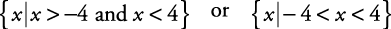## Absolute Value Inequalities

Remember, absolute value means distance from zero on a number line. | x| < 4 means that x is a number that is less than 4 units from zero on a number line (see Figure 1).

Figure 1. Less than 4 from zero.The solutions are the numbers to the right of –4 and to the left of 4 and could be indicated as| x| > 4 means that x is a number that is more than 4 units from zero on a number line (see Figure 2).

Back to Top
A18ACD436D5A3997E3DA2573E3FD792A